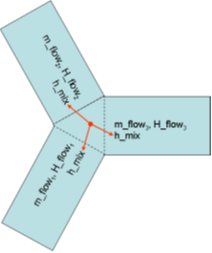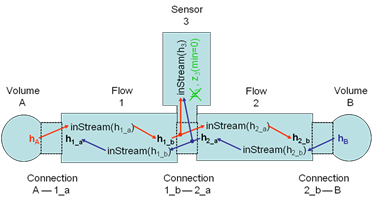# Appendix D Derivation of Stream Equations

This appendix contains a derivation of the equation for stream connectors from chapter 15.

# D.1 Reasons for avoiding the actual mixing enthalpy in connector definitions

Consider a connection set with n connectors. The mixing enthalpy is defined by the mass balance

 $0=\sum_{j=1}^{n}\dot{m}_{j}$

and the energy balance

 $0=\sum_{j=1}^{n}\dot{H}_{j}$

with

 $\dot{H}_{j}=\dot{m}_{j}\begin{cases}h_{mix}&\text{if \dot{m}_{j}>0}\\ h_{outflow,j}&\text{if \dot{m}_{j}<=0}\end{cases}$

Herein, mass flow rates are positive when entering models (exiting the connection set). The specific enthalpy represents the specific enthalpy inside the component, close to the connector, for the case of outflow. Expressed with variables used in the balance equations we arrive at:

 $h_{outflow,j}=\begin{cases}\frac{\dot{H}_{j}}{\dot{m}_{j}}&\text{if \dot{m}_{% j}<0}\\ \textrm{arbitrary}&\text{if \dot{m}_{j}>=0}\end{cases}$

While these equations are suitable for device-oriented modeling, the straightforward usage of this definition leads to models with discontinuous residual equations, which violates the prerequisites of several solvers for nonlinear equation systems. This is the reason why the actual mixing enthalpy is not modelled directly in the model equations. The stream connectors provide a suitable alternative.Figure D.1: Exemplary connection set with three connected components and a common mixing enthalpy

# D.2 Rationale for the formulation of the inStream() operator

For simplicity, the derivation of the inStream() operator is shown at hand of 3 model components that are connected together. The case for N connections follows correspondingly.

The energy and mass balance equations for the connection set for 3 components are (see above):

 $\begin{split}\displaystyle 0=&\displaystyle\dot{m}_{1}\cdot\begin{cases}h_{mix% }&\text{if \dot{m}_{1}>0}\\ h_{outflow,1}&\text{if \dot{m}_{1}<=0}\end{cases}\\ \displaystyle+&\displaystyle\dot{m}_{2}\cdot\begin{cases}h_{mix}&\text{if % \dot{m}_{2}>0}\\ h_{outflow,2}&\text{if \dot{m}_{2}<=0}\end{cases}\\ \displaystyle+&\displaystyle\dot{m}_{3}\cdot\begin{cases}h_{mix}&\text{if % \dot{m}_{3}>0}\\ h_{outflow,3}&\text{if \dot{m}_{3}<=0}\end{cases}\end{split}$ (D.1a) $0=\dot{m}_{1}+\dot{m}_{2}+\dot{m}_{3}$ (D.1b)

The balance equations are implemented using a max() operator in place of the piecewise expressions, taking care of the different flow directions:

 $\begin{split}\displaystyle 0=&\displaystyle\text{max}(\dot{m}_{1},0)h_{mix}-% \text{max}(-\dot{m}_{1},0)h_{outflow,1}\\ \displaystyle+&\displaystyle\text{max}(\dot{m}_{2},0)h_{mix}-\text{max}(-\dot{% m}_{2},0)h_{outflow,2}\\ \displaystyle+&\displaystyle\text{max}(\dot{m}_{3},0)h_{mix}-\text{max}(-\dot{% m}_{3},0)h_{outflow,3}\end{split}$ (D.2a) $\begin{split}\displaystyle 0=&\displaystyle\text{max}(\dot{m}_{1},0)-\text{max% }(-\dot{m}_{1},0)\\ \displaystyle+&\displaystyle\text{max}(\dot{m}_{2},0)-\text{max}(-\dot{m}_{2},% 0)\\ \displaystyle+&\displaystyle\text{max}(\dot{m}_{3},0)-\text{max}(-\dot{m}_{3},% 0)\end{split}$ (D.2b)

Equation (D.2a) is solved for $h_{mix}$

 $h_{mix}=\frac{\text{max}(-\dot{m}_{1},0)h_{outflow,1}+\text{max}(-\dot{m}_{2},% 0)h_{outflow,2}+\text{max}(-\dot{m}_{3},0)h_{outflow,3}}{\text{max}(\dot{m}_{1% },0)+\text{max}(\dot{m}_{2},0)+\text{max}(\dot{m}_{3},0)}$

Using (D.2b), the denominator can be changed to:

 $h_{mix}=\frac{\text{max}(-\dot{m}_{1},0)h_{outflow,1}+\text{max}(-\dot{m}_{2},% 0)h_{outflow,2}+\text{max}(-\dot{m}_{3},0)h_{outflow,3}}{\text{max}(-\dot{m}_{% 1},0)+\text{max}(-\dot{m}_{2},0)+\text{max}(-\dot{m}_{3},0)}$

Above it was shown that an equation of this type does not yield properly formulated model equations. In the streams concept we therefore decide to split the energy balance, which consists of different branches depending on the mass flow direction. Consequently, separate energy balances are the result; each valid for specific flow directions.

In a model governing equations have to establish the specific enthalpy of fluid leaving the model based on the specific enthalpy of fluid flowing into it. Whenever the mixing enthalpy is used in a model it is therefore the mixing enthalpy under the assumption of fluid flowing into said model.

We establish this quantity using a dedicated operator $inStream(h_{outflow,i})=h_{mix}$ $(\dot{m}_{i}>=0)$. This leads to three different incarnations of (n in the general case). This is illustrated in the figure below. For the present example of three components in a connection set, this means the following.

 $\displaystyle inStream(h_{outflow,1})$ $\displaystyle=$ $\displaystyle\frac{\text{max}(-\dot{m}_{2},0)h_{outflow,2}+\text{max}(-\dot{m}% _{3},0)h_{outflow,3}}{\text{max}(-\dot{m}_{2},0)+\text{max}(-\dot{m}_{3},0)}$ $\displaystyle inStream(h_{outflow,2})$ $\displaystyle=$ $\displaystyle\frac{\text{max}(-\dot{m}_{1},0)h_{outflow,1}+\text{max}(-\dot{m}% _{3},0)h_{outflow,3}}{\text{max}(-\dot{m}_{1},0)+\text{max}(-\dot{m}_{3},0)}$ $\displaystyle inStream(h_{outflow,3})$ $\displaystyle=$ $\displaystyle\frac{\text{max}(-\dot{m}_{1},0)h_{outflow,1}+\text{max}(-\dot{m}% _{2},0)h_{outflow,2}}{\text{max}(-\dot{m}_{1},0)+\text{max}(-\dot{m}_{2},0)}$

In the general case of a connection set with n components, similar considerations lead to the following.

 $inStream(h_{outflow,i})=\frac{\sum_{j=1,...,n;j\neq i}\text{max}(-\dot{m}_{j},% 0)h_{outflow,j}}{\sum_{j=1,...,n;j\neq i}\text{max}(-\dot{m}_{j},0)}$

# D.3 Special cases covered by the inStream() operator definition

## D.3.1 Stream connector is not connected (N=1):

For this case, the return value of the inStream() operator is arbitrary. Therefore, it is set to the outflow value.

## D.3.2 Connection of 2 stream connectors, one to one connections (N=2):

 $\displaystyle inStream(h_{outflow,1})$ $\displaystyle=$ $\displaystyle\frac{\text{max}(-\dot{m}_{2},0)h_{outflow,2}}{\text{max}(-\dot{m% }_{2},0)}=h_{outflow,2}$ $\displaystyle inStream(h_{outflow,2})$ $\displaystyle=$ $\displaystyle\frac{\text{max}(-\dot{m}_{1},0)h_{outflow,1}}{\text{max}(-\dot{m% }_{1},0)}=h_{outflow,1}$

In this case, inStream() is continuous (contrary to $h_{mix}$) and does not depend on flow rates. The latter result means that this transformation may remove nonlinear systems of equations, which requires that either simplifications of the form “a*b/a = b” must be provided, or that this case is treated directly.

## D.3.3 Connection of 3 stream connectors where one mass flow rate is identical to zero (N=3 and $\dot{m}_{3}=0$):

This case occurs, when a one-port sensor (like a temperature sensor) is connected to two connected components. For the sensor, the min attribute of the mass flow rate has to be set to zero (no fluid exiting the component via this connector). The suggested implementation results in the following equations, and as indicated the last formula can be simplified further by using $\dot{m}_{3}=0$:

 $\displaystyle inStream(h_{outflow,1})$ $\displaystyle=$ $\displaystyle h_{outflow,2}$ $\displaystyle inStream(h_{outflow,2})$ $\displaystyle=$ $\displaystyle h_{outflow,1}$ $\displaystyle inStream(h_{outflow,3})$ $\displaystyle=$ $\displaystyle\frac{\text{max}(-\dot{m}_{1},0)h_{outflow,1}+\text{max}(-\dot{m}% _{2},0)h_{outflow,2}}{\text{max}(-\dot{m}_{1},0)+\text{max}(-\dot{m}_{2},0)}$ $\displaystyle=$ $\displaystyle\begin{cases}h_{outflow,2}&\text{if \dot{m}_{1}>=0}\\ h_{outflow,1}&\text{if \dot{m}_{1}<0 and \dot{m}_{3}=0}\end{cases}$Figure D.3: Example series connection of multiple models with stream connectors

For the two components with finite mass flow rates (not the sensor), the properties discussed for two connected components still hold. The connection set equations reflect that the sensor does not any influence by discarding the flow rate of the latter. In several cases a non-linear equation system is removed by this transformation. However, inStream(..) results in a discontinuous equation for the sensor, which is consistent with modeling the convective phenomena only. The discontinuous equation is uncritical, if the sensor variable is not used in a feedback loop with direct feedthrough, since the discontinuous equation is then not part of an algebraic loop. Otherwise, it is advisable to regularize or filter the sensor signal.

## D.3.4 Connection of 3 stream connectors where two mass flow rates are positive (ideal splitting junction for uni-directional flow)

If uni-directional flow is present and an ideal splitter is modelled, the required flow direction should be defined in the connector instance with the “min” attribute (the “max” attribute could be also defined, however it does not lead to simplifications):

model m2
Fluidport c(m_flow(min=0));
...
end m2;

Consider the case of and all other mass flow rates positive (with the min attribute set accordingly). Connecting m1.c with m2.c and m3.c, such that

m2.c.m_flow.min = 0; // max(-m2.c.m_flow,0) = 0
m3.c.m_flow.min = 0; // max(-m3.c.m_flow,0) =

results in the following equation:

 $inStream(h_{outflow,1})=\frac{\text{max}(-\dot{m}_{2},0)h_{outflow,2}+\text{% max}(-\dot{m}_{3},0)h_{outflow,3}}{\text{max}(-\dot{m}_{2},0)+\text{max}(-\dot% {m}_{3},0)}=\frac{0}{0}$

The inStream() operator cannot be evaluated for a connector, on which the mass flow rate has to be negative by definition. The reason is that the value is arbitrary, which is why it is defined as follows.

 $inStream(h_{outflow,1}):=h_{outflow,1}$

For the remaining connectors the inStream() operator reduces to a simple result.

 $\displaystyle inStream(h_{outflow,2})$ $\displaystyle=\frac{\text{max}(-\dot{m}_{1},0)h_{outflow,1}+\text{max}(-\dot{m% }_{3},0)h_{outflow,3}}{\text{max}(-\dot{m}_{1},0)+\text{max}(-\dot{m}_{3},0)}=% h_{outflow,1}$ $\displaystyle inStream(h_{outflow,3})$ $\displaystyle=\frac{\text{max}(-\dot{m}_{1},0)h_{outflow,1}+\text{max}(-\dot{m% }_{2},0)h_{outflow,2}}{\text{max}(-\dot{m}_{1},0)+\text{max}(-\dot{m}_{2},0)}=% h_{outflow,1}$

Again, the previous non-linear algebraic system of equations is removed. This means that utilizing the information about uni-directional flow is very important.

To summarize, if all mass flow rates are zero, the balance equations for stream variables (D.1) and for flows (D.2) are identically fulfilled. In such a case, any value of h_mix fulfills (D.1), i.e., a unique mathematical solution does not exist. This specification only requires that a solution fulfills the balance equations. Additionally, a recommendation is given to compute all unknowns in a unique way, by providing an explicit formula for the inStream operator. Due to the definition, that only flows where the corresponding “min” attribute is neither zero nor positive enter this formula, a meaningful physcial result is always obtained, even in case of zero mass flow rate. As a side effect, non-linear equation systems are automatically removed in special cases, like sensors or uni-directional flow, without any symbolic transformations (no equation must be analyzed; only the “min”-attributes of the corresponding flow variables).